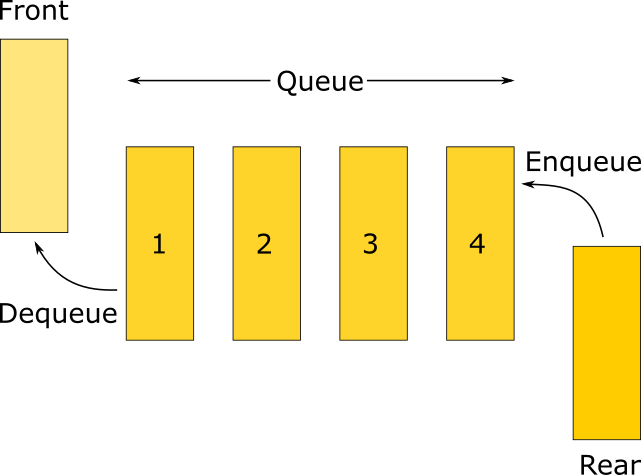Before Talking specifically about Java Queue let’s first understand what Queue is in General?

A Queue is a linear Data Structure that follows FIFO(First in First out mechanism for input/output). Here is a Diagrammatic representation of how a Queue works!The Queue interface present in java.util package and extends the Collection interface. It is an ordered list of objects with its use limited to insert elements at the end of the list and deleting elements from the start of the list.
Being an interface the queue needs a concrete class for the declaration and the most common classes are the PriorityQueue and LinkedList in Java. It is to be noted that both the implementations are not thread-safe. PriorityBlockingQueue is one alternative implementation if the thread-safe implementation is needed.## How to Use Java Queue?

Since Queue is an interface, objects cannot be created of the type queue. We always need a class that extends this list in order to create an object. And also, after the introduction of Generics in Java 1.5, it is possible to restrict the type of object that can be stored in the Queue. This type-safe queue can be defined as:

``Queue<Object> queue = new PriorityQueue<Object> ()``

Note how <Object> is specified two times. We can use any Class to define the type during declaration. That means we can use predefined classes as well as classes of our own! That’s the power of generics. Here we are going to use PriorityQueue to demonstrate operations on queue in Java. The same can be done using a linked list. In nutshell, both are lists but Priority Queue assigns weight to its input and arranges them in ascending order during insertion whereas linked list follows the order of insertion.Basically, we can perform two functions on a Queue. Let’s take a look at them one by one.

### ENQUEUE

In Java, we don’t have the enqueue method defined with the name add(). Here’s how we use it.

``````import java.util.PriorityQueue;
import java.util.Queue;

public class QueueExample {
public static void main(String[] args) {
Queue queue = new PriorityQueue<> ();
System.out.println(queue+"");
}
}``````

OUTPUT

``[1, 2, 3]``

### DEQUEUE

Here Java offers two methods we can use –

1. poll()
2. remove()

Both of them return the head element of the Queue – following FIFO. The remove and poll methods differ in their behavior only when the queue is empty. Under these circumstances, remove throws NoSuchElementException, while poll returns null.

#### poll()

``````import java.util.PriorityQueue;
import java.util.Queue;

public class QueueExample {
public static void main(String[] args) {
Queue queue = new PriorityQueue<> ();
queue.add(1); // first enqueue
queue.add(2); // second enqueue
queue.add(3); // third enqueue
System.out.println("Queue before poll:"+queue);
System.out.println("dequeue: "+queue.poll());
System.out.println("Queue after poll:"+queue);
}
}``````

OUTPUT

``````Queue before poll:[1, 2, 3]
dequeue: 1
Queue after poll:[2, 3]``````

#### remove()

``````import java.util.PriorityQueue;
import java.util.Queue;

public class QueueExample {
public static void main(String[] args) {
Queue queue = new PriorityQueue<> ();
queue.add(1); // first enqueue
queue.add(2); // second enqueue
queue.add(3); // third enqueue
System.out.println("Queue before poll:"+queue);
System.out.println("dequeue: "+queue.remove());
System.out.println("Queue after poll:"+queue);
}
}``````

OUTPUT

``````Queue before poll:[1, 2, 3]
dequeue: 1
Queue after poll:[2, 3]``````

As you can see both the code produces the same output.

## More On Java Queue Methods

In JAVA we have many more functions available to use with Queue. For instance –

### Java size() method

Returns the size of the queue.

``````import java.util.PriorityQueue;
import java.util.Queue;

public class QueueExample {
public static void main(String[] args) {
Queue queue = new PriorityQueue<> ();
queue.add(1); // first enqueue
queue.add(2); // second enqueue
queue.add(3); // third enqueue
System.out.println("Queue size: "+queue.size());
}
}
``````

OUTPUT

``Queue size: 3``

### peek()

Returns the head element in Queue but does not remove it.

``````import java.util.PriorityQueue;
import java.util.Queue;

public class QueueExample {
public static void main(String[] args) {
Queue queue = new PriorityQueue<> ();
queue.add(1); // first enqueue
queue.add(2); // second enqueue
queue.add(3); // third enqueue
System.out.println("Queue before poll:"+queue);
System.out.println("Queue after poll:"+queue);
}
}
``````

OUTPUT

``````Queue before poll:[1, 2, 3]
Queue after poll:[1, 2, 3]``````

### Java queue element() method

element() method, just like the peek() method returns the head element but does not remove it. They differ from one another in precisely the same fashion as remove and poll: if the queue is empty, the element throws NoSuchElementException while the peek returns false.

``````import java.util.PriorityQueue;
import java.util.Queue;

public class QueueExample {
public static void main(String[] args) {
Queue queue = new PriorityQueue<> ();
queue.add(1); // first enqueue
queue.add(2); // second enqueue
queue.add(3); // third enqueue
System.out.println("Queue before poll:"+queue);
System.out.println("Queue after poll:"+queue);
}
}``````

OUTPUT

``````Queue before poll:[1, 2, 3]
Queue after poll:[1, 2, 3]``````

#### contains(Object o)

Returns true if the element is present in the queue, false if not.

``````import java.util.PriorityQueue;
import java.util.Queue;

public class QueueExample {
public static void main(String[] args) {
Queue queue = new PriorityQueue<> ();
queue.add(1); // first enqueue
queue.add(2); // second enqueue
queue.add(3); // third enqueue
System.out.println("Queue before poll:"+queue);
System.out.println("Does the Queue contain value 1?: "+queue.contains(1));
}
}``````

#### OUTPUT

``````Queue before poll:[1, 2, 3]
Does the Queue contain value 1?: true``````